# ریخت شناسی در پردازش تصاویر دیجیتال

## ریخت شناسی در پردازش تصاویر دیجیتال

یکی از مقوله های جالب توجه در پردازش تصویر، عملگرهای ریخت شناسی یا همان عملگرهای مورفولوژی است. قصد نوشتن یک درسنامه در باب این موضوع داشتم که دیدم متنی به زیبایی متن زیر که اقتباس شده از فصل یازدهم از کتاب زیر است، نمی توان به رشته ی تحریر در آورد.

• Nick Efford. Digital Image Processing: A Practical Introduction Using JavaTM. Pearson Education, 2000.

به همین دلیل، بدون دخل و تصرف آنرا در ادامه آوردم. در اولین فرصت، ترجمه ی آن نیز در متن قرارداده خواهد شد.

Binary images may contain numerous imperfections. In particular, the binary regions produced by simple thresholding are distorted by noise and texture. Morphological image processing pursues the goals of removing these imperfections by accounting for the form and structure of the image. These techniques can be extended to greyscale images.

## Basic concepts

Morphological image processing is a collection of non-linear operations related to the shape or morphology of features in an image. According to Wikipedia, morphological operations rely only on the relative ordering of pixel values, not on their numerical values, and therefore are especially suited to the processing of binary images. Morphological operations can also be applied to greyscale images such that their light transfer functions are unknown and therefore their absolute pixel values are of no or minor interest.

Morphological techniques probe an image with a small shape or template called a structuring element. The structuring element is positioned at all possible locations in the image and it is compared with the corresponding neighbourhood of pixels. Some operations test whether the element “fits” within the neighbourhood, while others test whether it “hits” or intersects the neighbourhood: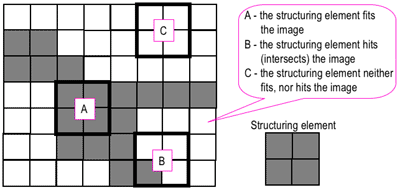Probing of an image with a structuring element
(white and grey pixels have zero and non-zero values, respectively).

A morphological operation on a binary image creates a new binary image in which the pixel has a non-zero value only if the test is successful at that location in the input image.

The structuring element is a small binary image, i.e. a small matrix of pixels, each with a value of zero or one:

• The matrix dimensions specify the size of the structuring element.
• The pattern of ones and zeros specifies the shape of the structuring element.
• An origin of the structuring element is usually one of its pixels, although generally the origin can be outside the structuring element.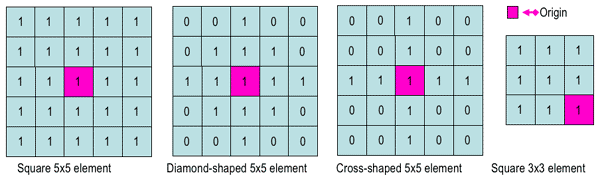Examples of simple structuring elements.

A common practice is to have odd dimensions of the structuring matrix and the origin defined as the centre of the matrix. Stucturing elements play in moprphological image processing the same role as convolution kernels in linear image filtering.

When a structuring element is placed in a binary image, each of its pixels is associated with the corresponding pixel of the neighbourhood under the structuring element. The structuring element is said to fit the image if, for each of its pixels set to 1, the corresponding image pixel is also 1. Similarly, a structuring element is said to hit, or intersect, an image if, at least for one of its pixels set to 1 the corresponding image pixel is also 1.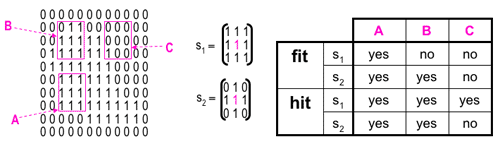Fitting and hitting of a binary image with structuring elements s1 and s2.

Zero-valued pixels of the structuring element are ignored, i.e. indicate points where the corresponding image value is irrelevant.

## Fundamental operations

More formal descriptions and examples of how basic morphological operations work are given in the Hypermedia Image Processing Reference (HIPR) developed by Dr. R. Fisher et al. at the Department of Artificial Intelligence in the University of Edinburgh, Scotland, UK.

### Erosion and dilation

The erosion of a binary image f by a structuring element s (denoted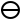s) produces a new binary image g =s with ones in all locations (x,y) of a structuring element’s origin at which that structuring element s fits the input image f, i.e. g(x,y) = ۱ is s fits f and 0 otherwise, repeating for all pixel coordinates (x,y).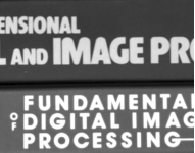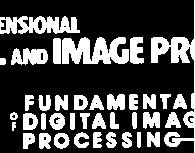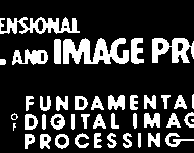Greyscale image Binary image by thresholding Erosion: a 2×۲ square structuring element http://documents.wolfram.com/applications/digitalimage/UsersGuide/Morphology/ImageProcessing6.3.html

Erosion with small (e.g. 2×۲ – ۵×۵) square structuring elements shrinks an image by stripping away a layer of pixels from both the inner and outer boundaries of regions. The holes and gaps between different regions become larger, and small details are eliminated: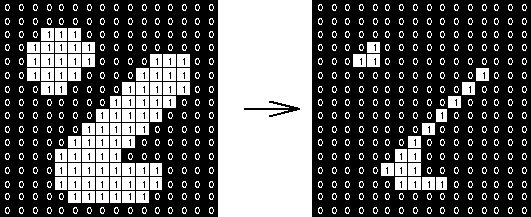Erosion: a 3×۳ square structuring element
(www.cs.princeton.edu/~pshilane/class/mosaic/).

Larger structuring elements have a more pronounced effect, the result of erosion with a large structuring element being similar to the result obtained by iterated erosion using a smaller structuring element of the same shape. If s1 and s2 are a pair of structuring elements identical in shape, with s2 twice the size of s1, thens2 ≈ (s1s1.

Erosion removes small-scale details from a binary image but simultaneously reduces the size of regions of interest, too. By subtracting the eroded image from the original image, boundaries of each region can be found: b = f − (fs ) where f is an image of the regions, s is a 3×۳ structuring element, and b is an image of the region boundaries.

The dilation of an image f by a structuring element s (denoted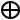s) produces a new binary image g =s with ones in all locations (x,y) of a structuring element’s orogin at which that structuring element s hits the the input image f, i.e. g(x,y) = ۱ if s hits f and 0 otherwise, repeating for all pixel coordinates (x,y). Dilation has the opposite effect to erosion — it adds a layer of pixels to both the inner and outer boundaries of regions.Binary image Dilation: a 2×۲ square structuring element http://documents.wolfram.com/applications/digitalimage/UsersGuide/Morphology/ImageProcessing6.3.html

The holes enclosed by a single region and gaps between different regions become smaller, and small intrusions into boundaries of a region are filled in: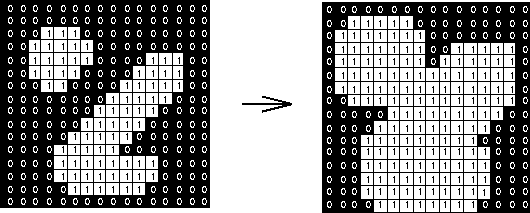Dilation: a 3×۳ square structuring element
(www.cs.princeton.edu/~pshilane/class/mosaic/).

Results of dilation or erosion are influenced both by the size and shape of a structuring element. Dilation and erosion are dual operations in that they have opposite effects. Let f c denote the complement of an image f, i.e., the image produced by replacing 1 with 0 and vice versa. Formally, the duality is written ass = f csrot

where srot is the structuring element s rotated by 180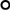. If a structuring element is symmetrical with respect to rotation, then srot does not differ from s. If a binary image is considered to be a collection of connected regions of pixels set to 1 on a background of pixels set to 0, then erosion is the fitting of a structuring element to these regions and dilation is the fitting of a structuring element (rotated if necessary) into the background, followed by inversion of the result.

### Compound operations

Many morphological operations are represented as combinations of erosion, dilation, and simple set-theoretic operations such as the complement of a binary image:

f c(x,y) = ۱ if f(x,y) = ۰, and f c(x,y) = ۰ if f(x,y) = ۱,

the intersectionh = f ∩ g of two binary images f and g:

h(x,y) = ۱ if f(x,y) = ۱ and g(x,y) = ۱, and h(x,y) = ۰ otherwise,

and the unionh = f ∪ g of two binary images f and g:

h(x,y) = ۱ if f(x,y) = ۱ or g(x,y) = ۱, and h(x,y) = ۰ otherwise: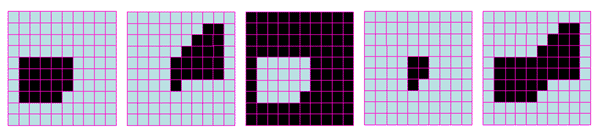Set operations on binary images: from left to right: a binary image f,
a binary image g, the complement f c of f, the intersection f ∩ g, and the union f ∪ g.

The opening of an image f  by a structuring element s (denoted bys) is an erosion followed by a dilation:s = (ss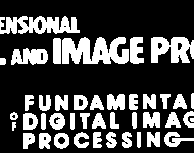Binary image Opening: a 2×۲ square structuring element http://documents.wolfram.com/applications/digitalimage/UsersGuide/Morphology/ImageProcessing6.3.html

Opening is so called because it can open up a gap between objects connected by a thin bridge of pixels. Any regions that have survived the erosion are restored to their original size by the dilation: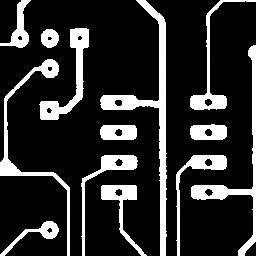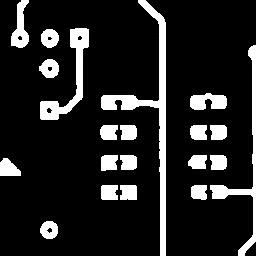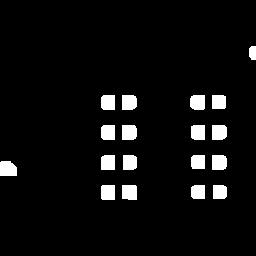Binary image f fs (۵×۵ square) fs (۹×۹ square) Results of opening with a square structuring element (www.mmorph.com/html/morph/mmopen.html/).

Opening is an idempotent operation: once an image has been opened, subsequent openings with the same structuring element have no further effect on that image:

(s)s) =s.

The closing of an image f  by a structuring element s (denoted by f • s) is a dilation followed by an erosion:

f • s = (srotsrotBinary image Closing: a 2×۲ square structuring element http://documents.wolfram.com/applications/digitalimage/UsersGuide/Morphology/ImageProcessing6.3.html

In this case, the dilation and erosion should be performed with a rotated by 180structuring element. Typically, the latter is symmetrical, so that the rotated and initial versions of it do not differ.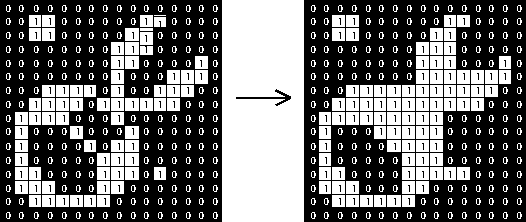Closing with a 3×۳ square structuring element
(www.cs.princeton.edu/~pshilane/class/mosaic/).

Closing is so called because it can fill holes in the regions while keeping the initial region sizes. Like opening, closing is idempotent: (f • s) • s = f • s, and it is dual operation of opening (just as opening is the dual operation of closing):

f • s = (f cs)c;s = (f c • s)c.

In other words, closing (opening) of a binary image can be performed by taking the complement of that image, opening (closing) with the structuring element, and taking the complement of the result.

The hit and miss transform (see also HIPS2 web page) allows to derive information on how objects in a binary image are related to their surroundings. The operation requires a matched pair of structuring elements, {s1s2}, that probe the inside and outside, respectively, of objects in the image:

f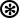{s1s2} = (fs1) ∩ (f cs2).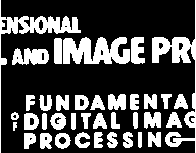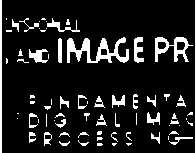Binary image Hit and miss transform: an elongated 2×۵ structuring element http://documents.wolfram.com/applications/digitalimage/UsersGuide/Morphology/ImageProcessing6.3.html

A pixel belonging to an object is preserved by the hit and miss transform if and only if s1 translated to that pixel fits inside the object AND s2 translated to that pixel fits outside the object. It is assumed that s1 and s2 do not intersect, otherwise it would be impossible for both fits to occur simultaneously.

It is easier to describe it by considering s1 and s2 as a single structuring element with 1s for pixels of s1 and 0s for pixels of s2; in this case the hit-and-miss transform assigns 1 to an output pixel only if the object (with the value of 1) and background (with the value of 0) pixels in the structuring element exactly match object (1) and background (0) pixels in the input image. Oterwise that pixel is set to the background value (0).

The hit and miss transform can be used for detecting specific shapes (spatial arrangements of object and background pixel values) if the two structuring elements present the desired shape, as well as for thinning or thickening of object linear elements.

Morphological filtering

of a binary image is conducted by considering compound operations like opening and closing as filters. They may act as filters of shape. For example, opening with a disc structuring element smooths corners from the inside, and closing with a disc smooths corners from the outside. But also these operations can filter out from an image any details that are smaller in size than the structuring element, e.g. opening is filtering the binary image at a scale defined by the size of the structuring element. Only those portions of the image that fit the structuring element are passed by the filter; smaller structures are blocked and excluded from the output image. The size of the structuring element is most important to eliminate noisy details but not to damage objects of interest.

## References

These lecture notes follow Chapter 11 “Morphological image processing” of the textbook

• Nick Efford. Digital Image Processing: A Practical Introduction Using JavaTM. Pearson Education, 2000.

with extra examples and teaching materials taken mostly, with corresponding references, from the Web.

0/5 ( 0 نظر )